﻿ On the Analytic Curve of C<SUP>2</SUP> which is not Omitted by Every Fatou-Bieberbach Domain

On the Analytic Curve of C2 which is not Omitted by Every Fatou-Bieberbach DomainOPEN ACCESSPEER-REVIEWED

On the Analytic Curve of C2 which is not Omitted by Every Fatou-Bieberbach Domain

YUKINOBU ADACHIYukinobu Adachi, Kurakuen, Nishinomiya, Hyogo, Japan

Abstract

Let C be an irreducible (may be transendental) analytic curve whose genus is geater than 1. Then every Fatou-Bieberbach domain does not omit C.

• ADACHI, YUKINOBU. "On the Analytic Curve of C2 which is not Omitted by Every Fatou-Bieberbach Domain." American Journal of Mathematical Analysis 3.1 (2015): 19-20.
• ADACHI, Y. (2015). On the Analytic Curve of C2 which is not Omitted by Every Fatou-Bieberbach Domain. American Journal of Mathematical Analysis, 3(1), 19-20.
• ADACHI, YUKINOBU. "On the Analytic Curve of C2 which is not Omitted by Every Fatou-Bieberbach Domain." American Journal of Mathematical Analysis 3, no. 1 (2015): 19-20.

 Import into BibTeX Import into EndNote Import into RefMan Import into RefWorks

1. Introduction

We call that a domain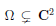is a Fatou-Bieberbach domain if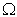is biholomorphic to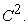We call that an irreducible algebraic curve A of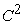is hyperbolic when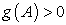or else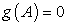and the set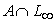consists more than two different points, where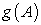is the genus of A and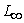is the line at infinity.

In the previous paper , we proved the case A (theorem 2.1). In this paper, every Fatou-Bieberbach domain does not omit C which is the same in Abstract (Theorem 3.12). Moreover we prove that the property that an analytic curve of C2 does not omit every Fatou-Bieberbach domain is unchanged one by a transformation of Aut(C2) (Theorem 3.7). Therefore every Fatou-Bieberbach domain does not omit T(A) with T Aut(C2). And we prove also that there is an algebraic type curve of C2 which is isomorphic to an algebraic curve of any topological type such that it can not be transformed to an algebraic curve by any T Aut(C2) (Proposition 3.3).

2. Preliminary

Theorem 2.1 (Theorem 2.1 in ). Let A be a hyperbolic algebraic curve of C2. Then every Fatou-Bieberbach domain Ω does not omit A.

Remark 2.2 (Theorem 2.2 in ). Let Ω0 be every Fatou-Bieberbach domain in C2 arising from a polynomial automorphism, namely a polynomial basin. Then Ω0 does not omit every algebraic curve of C2.

Theorem 2.3 (Theorem 4.1 in Buzzard and Fornaess ). Let X be an arbitrary analytic curve of C2. Then there is a Fatou-Bieberbach domain Ω with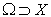and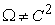where some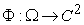is biholomorphic such that every nonconstant holomorphic map f : C C2 intersects with Φ(X) at in_nite points and C2 Φ(X) is Kobayashi hyperbolic.

Example 2.4 (Example 9.7 in ). There is a Fatou-Bieberbach domain Ω0 such that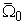namely the closure of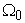omits an algebraic complex line L.

Proposition 2.5 (Proposition 3.1 in ). For some transendental complex line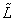such that there is a Fatou-Bieberbach domain Ω0 with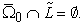From Theorem 2.3, Example 2.4 and Proposition 2.5, following theorem is easy to see.

Theorem 2.6. Let C be an analytic curve of C2. Then every Fatou-Bieberbach domain does not omit C or some (not all) Fatou-Bieberbach do-main Ω0 omits C.

3. Conclusion

Proposition 3.1. Let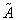be a transendental hyperbolic curve of C2 which is isomorphic to a hyperbolic algebraic curve A. Ifis transformed to an alge-braic hyperbolic curve A of C2 by T Aut(C2), then every Fatou-Bieberbach domain does not omit.

Proof. We assume that there is a Fatou-Bieberbach domain Ω with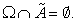Then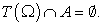and T(Ω) is biholomorphic to C2 by T Φ, where Φ is a biholomorphic map of Ω to C2. Therefore T(Ω) is a Fatou-Bieberbach domain and it contradicts to Theorem 2.1.

Proposition 3.2. Let X and Ω be the same of Theorem 2.3. Then Φ(X) can not be transformed to any algebraic curve A which is hyperbolic or non by any T Aut(C2).

Proof. We assume that Φ(X) is transformed to an algebraic curve A by some T Aut(C2). As T(Ω) is a Fatou-bieberbach domain with T Φ(X) = A. Let f : C C2 be a map to an algebraic complex line. Then (T Φ(X)) f(C) is a finite set of points at most and Φ(X) (T1 f(C)) is also. It is a contradiction to Theorem 2.3.

Proposition 3.3 (cf. Proposition 3.12 in ). There is a transcendental analytic curve of C2 which is isomorphic to an algebraic curve of any topo-logical type, that is an algebraic type curve of any type, such that it can not be transformed to an algebraic curve by any T Aut(C2). If we take X an algebraic type curve of any topological type, Φ(X) which is the same notation of Theorem 2.3 is such one.

Proposition 3.4. Let X, Ω and Φ be the same of Theorem 2.3. Then every Fatou-Bieberbach domain Ωdoes not omit Φ(X).

Proof. If some Ωomits Φ(X), Φ1 : C2 Ω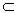C2Φ(X), where Φis a biholomorphic map of Ωto C2. Since C2Φ(X) is Kobayashi hyperbolic, Φ1 is a constant map. It is a contradiction.

Proposition 3.5. Let C be an analytic curve of C2 which is transformed by T Aut(C2) to some analytic curve Cof C2 which does not omit every Fatou-Bieberbach domain. Then every Fatou-Bieberbach domain does not omit C.

Proof. We assume that there is a Fatou-Bieberbach domain Ω0 with Ω0 ∩ C =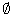. Then T(Ω0) ∩ T(C) =. Since T(Ω0) is a Fatou-Bieberbach domain which omits C′ = T(C). It contradicts to the property of C.

Corollary 3.6. Let C be an analytic curve of C2 which does not omit every Fatou-Bieberbach domain. Then T(C) with every T Aut(C2) does not omits every Fatou-Bieberbach domain.

Proof. Since T1 ◦ T(C) = C, T(C) does not omit every Fatou-Bieberbach domain also by Proposition 3.5.

From Proposition 3.5 and Corollary 3.6 it is easy to see the following theorem.

Theorem 3.7. The property that the analytic curve of C2 does not omit every Fatou-Bieberbach domain is unchanged one by a transformation of Aut(C2).

From Proposition 3.4 and Theorem 3.7 it is easy to see the following corollary.

Corollary 3.8. Let C be an analytic curve of C2 which is transformed by some T Aut(C2) to Φ(X) where X, Ω and Φ are the same of Proposition 3.4. Then every Fatou-Bieberbach domain Ωdoes not omit C.

Problem 3.9. Is therewhich is not transformed to an algebraic hyperbolic curve A of C2 or Φ(X), which is the same notation of Theorem 2.3, by some T Aut(C2)?

Proposition 3.10. Let C be an irreducible analytic curve of C2 with g(C) > 1. Then D = C2 C is not biholomorphic to C2, that is, D is not a Fatou-Bieberbach domain.

Proof. We assume that there is a biholomorphic map Φ of D to C2(x, y) such as Φ : x = ξ(z,w), y = η(z,w) where (z,w) D. Let P(x, y) be a nonconstant primitive polynomial, that is all level curve of {P(x, y) = α} is irreducible except at most finite number of α1, · · · , αn. Then P(ξ(z,w), η(z,w))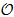(D). If C is an essential singular curve of P(ξ, η), every level curve of P(ξ, η) can not be analytically continued to every point of C except at most one value α0 by well known Thullen’s theorem.

Since {P(ξ, η) = α, α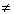α0, α1, · · · , αn} is considered as a Riemann surface R0 = R − {p1, · · · , pm} where R is a compact Riemann surface, that is an algebraic type Riemann surface such as π : R0 → {P(ξ, η) = α}C2 is the normalization. And at some pi ∈ {p1, · · · , pm} the cluster set of π in C2 is C. Because it is a pseudoconcave set of C2 by Theorem 3.4 in  and it is a degeneration locus of Kobayashi pseudodistance by Theorem 3.6 in . It is a contradiction to g(C) > 1 by Theorem 4 in .

Therefore C is not an essential singular curve of P(ξ, η), that is, P(ξ, η) is at most meromorphically continued to C. Since P(x, y)(C2), P(ξ, η) is holomorphically continued to C. We set such function as F(z,w).

Since every level curve of F(z,w) is holomorphically isomorphic to algebraic type Riemann surface and an analytic cuve of C2, F(z,w) is an algebraic type entire function of Nishino’s sence , namely in the class (A). Then by principal theorem of , F = φ Q T where φ is a polynomial function of one complex variable because P(x, y) is primitive, Q is a primitive polynomial and T Aut(C2).

Then T|D = Φ. It contradicts to the assumption. Then D is not biholomorphic to C2.

Proposition 3.11. Let C be an irreducible analytic curve of C2 such that D = C2 C is not a Fatou-Bieberbach domain. Then every subdomain of Dof D is not a Fatou-Bieberbach domain.

Proof. If Dis a Fatou-Bieberbach domain, there is a biholomorphic map Φ of C2 to D′. Let I be an inclusion map of D′ to D and {f = 0} = C where f ∈(C2). Since g = f ◦ I ◦ Φ is an entire function of C2 and g ≠ 0. There is a complex line L of C2 and g|L is considered as a transendental entire function of C, it is a contradiction by little Picard theorem because I ◦ Φ|L is an one to one map.

From Proposition 3.10 and 11, following theorem is easy to see.

Theorem 3.12. Let C be the same of Proposition 3.10. Then every Fatou-Bieberbach domain does not omit C.

References

  Y. Adachi, A generalization of the big Picard theorem, Kodai Math. J., 18(1995), 408-424.In article CrossRef  Y. Adachi, Remarks about Fatou-Bieberbach domains and algebraic or non-algebraic curves in C2, Far East J. Math. Soc. (FJMS), 34 (2009), 369-376.In article  Y. Adachi and Masakazu Suzuki, Degeneracy points of the Kobayashi pseudodistances on complex manifolds, Proceedings of Symposia in Pure Math., 52 (1991), Part 2, 41-51.In article  G. T. Buzzard and J. E. Fornaess, An embedding of C in C2 with hyperbolic complement, Math. Ann. 306 (1996), 539-546.In article CrossRef  T. Nishino, Nouvelles recherches sur les fonctions entières de plusieurs variables conplexes (V) Fonctions qui se réduisent aux polynômes, J. Math. Kyoto Univ., 15 (1975), 527-553.In article  J. P. Rosay and W. Rudin, Holomorphic maps from Cn to Cn, Trans. A. M. S., 310 (1988), 47-86.In article